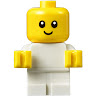D

#### Greatest K to 12 Math Problem

27 viewed last edited 5 years agoAnonymous
0

The letters A, B, C and D represent the number of cows in the respective square. The numbers W, X, Y and Z on the bridges represent the sum of the squares they are connecting. For e.g. W = A + D.

How many solutions are possible where all numbers are single digit numbers; and there are no repetitions?Some people consider this the "The Greatest K to 12 Math Problem Ever"

https://medium.com/q-e-d/the-greatest-k-to-12-math-problem-ever-4bf701dd04b9Jonathan Le
1

Two possible solutions are:

2(A) - 6(X) - 4(B)

9(W) -        - 5(Y)

7(D) - 8(Z) - 1(C)

and

2(A) - 8(X) - 6(B)

5(W) -        - 7(Y)

3(D) - 4(Z) - 1(C)

Other analysis: We need to pick 8 numbers from the 9 possible. So, we have to discard 1 of the numbers. There are 9 ways to discard one number, giving a max of 9 solutions. Obviously, this is an upper bound as it is highly likely that 1 needs to be picked.

Other thoughts:

How many even numbers can be on the squares? There are 4 even numbers, and if two even numbers are adjacent to each other the bridge (sum of the number on the squares) will also be even.

So, it is not possible that all 4 even numbers are on the squares. This doesn't leave any even numbers for the bridge. 0 zero solutions where the squares have all even numbers.

It is also not possible to have 3 even numbers on the squares. 2 sets of evens have to be on adjacent squares - needing a total of 5 even numbers. 0 zero solutions where the squares have 3 even numbers.

So, the squares contain 1 even number of 2 even numbers. In both the solutions above, the squares contain 2 even numbers.

A solution with 1 even number on the squares:

2(A) - 5(X) - 3(B)

9(W) -        - 4(Y)

7(D) - 8(Z) - 1(C)

With 1 even number on the squares, 2 and 1 cannot be adjacent to each other. This will quickly make the other numbers on the squares too big. I feel there can only one possible solution with one even number on the squares. 1 solution where the squares have 1 even number.

We saw 2 solutions where there are 2 even numbers on the squares. Can there be more solutions?

The 2 even numbers have to be adjacent to each other. If the even numbers are not adjacent to each other, we need 6 odd numbers. But this is not possible as we have only 5 odd numbers.

The 2 solutions with 2 evens have:

• 2 and 4 next to each other; and
• 2 and 6 next to each other

There can be no solutions with 2 and 8 on adjacent squares - we need a 10 on the bridge.

There can be no solutions with 4 and 6 on adjacent squares - we need a 10 on the bridge.

With 2 and 4 on adjacent squares, we found one solution. Can there be more solutions?

Quite an interesting problem. I feel younger children can guess and find solutions. Older children can analyse and come up with better answers.